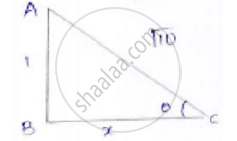Share

# In the Following, Trigonometric Ratios is Given. Find the Values of the Other Trigonometric Ratios. Cosec Theta = Sqrt10 - CBSE Class 10 - Mathematics

#### Question

In the following, trigonometric ratios are given. Find the values of the other trigonometric ratios.

cosec theta = sqrt10

#### Solution

consider a right-angled Δle ABC, we getLet x be the adjacent side.

By applying Pythagoras theorem

𝐴𝐶2 = 𝐴𝐵2 + 𝐵𝐶2

(sqrt10)^2 = 1^2 + x^2

x2 = 10 − 1 = 9

x = 3

sin theta = 1/cosec theta = 1/sqrt10

cos theta = "adjacent"/"hypotenuse" = 3/sqrt10

tan theta = "opposite sides"/"adjacebt side" = 1/3

sec theta = 1/cos theta = sqrt10/3

cot theta = 1/tan theta = (1/1)/3 = 3

Is there an error in this question or solution?

#### APPEARS IN

Solution In the Following, Trigonometric Ratios is Given. Find the Values of the Other Trigonometric Ratios. Cosec Theta = Sqrt10 Concept: Trigonometric Ratios.
S